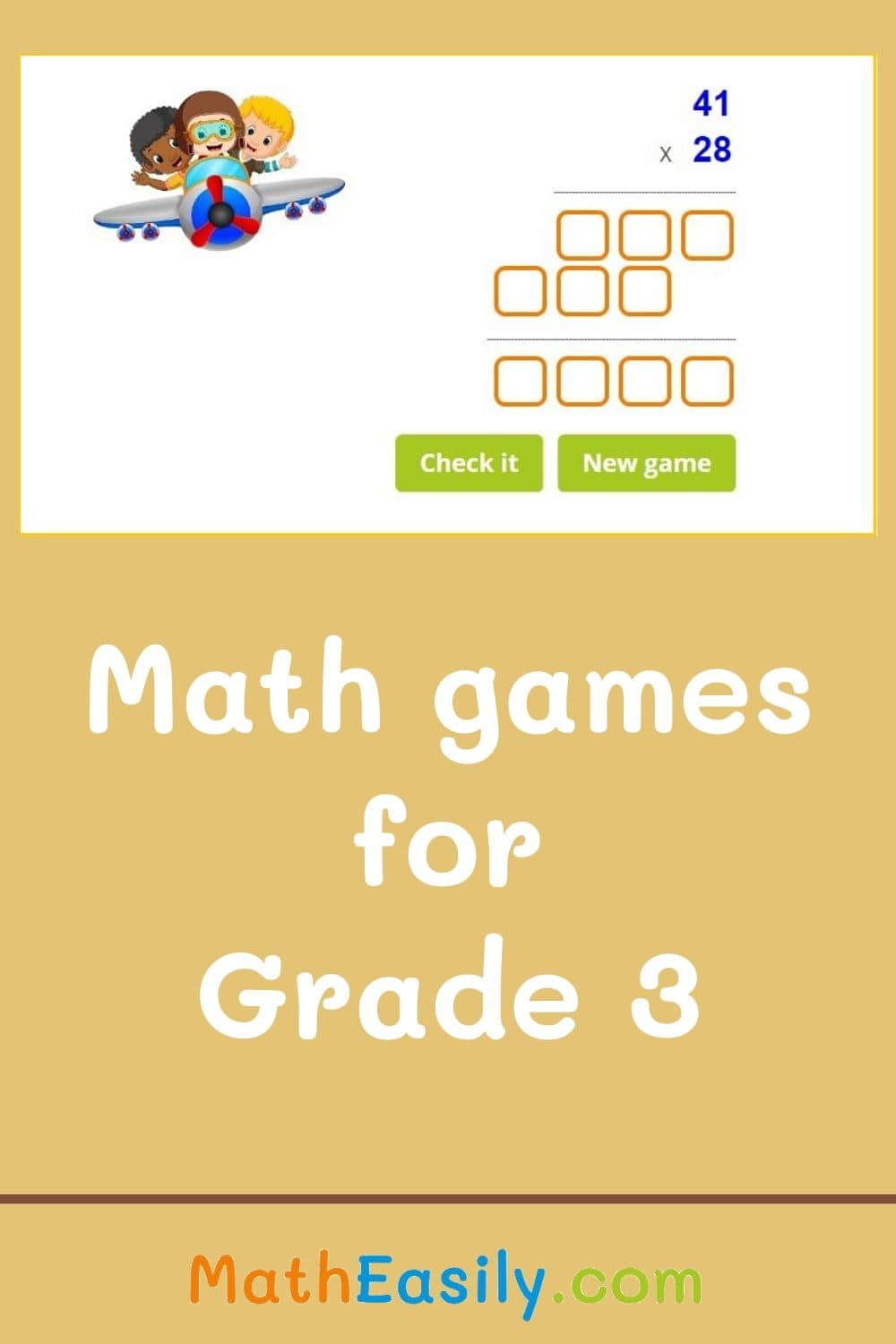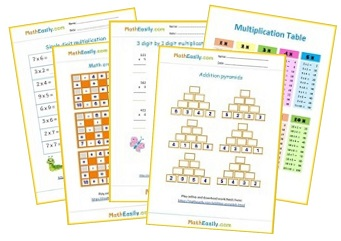﻿ 100+ Free Math Games for Grade 3 ONLINE Practice

Page No. 1051

Online Maths Games > 3rd Grade Math

# Online Math Games for Grade 3 FREE

Play free math games for grade 3 online. Learn addition, subtraction, multiplication, division and much more. Use our interactive 3rd grade math games in the classroom or for home practice.

All these online math games for 3rd grade contain also printable third grade math games and worksheets on which kids can practice a certain skill. Find all our 3rd grade math worksheets here.

## Free Math Games for Grade 3 ONLINE

### Multiplication dice game

Roll a pair of dice, multiply 2 numbers, check your answer. Play multiplication games with a dice.
Play now

### Adding and subtracting within 100

Play now

Play interactive ´2 digit addition´ game. Move the digits on the left side into the correct boxes.
Play now

### Math picture puzzles

Play interactive picture puzzles. Learn addition, subtraction and multiplication. Solve the picture equations.
Play now

### 2 digit subtraction online games

Practice 2 digit subtraction with regrouping and without regrouping: online games and worksheets.
Play now

Play now

### Fraction flash cards

Learn fractions easily with these 3rd grade math games. Fraction flash cards use visual aids (circles or fraction bars) to help students understand the concept of fractions.
Play now

### Division puzzles

Learn to divide with these free math games for 3rd graders. Math division puzzles are a great method how students learn division easily. As kids complete the division tasks, they gradually reveal pictures of animals.
Play now

### 3 digit addition games online

You can choose between 2 options: 3 digit addition games with or without regrouping. Play learning math games for 3rd graders online in the classroom or at home.
Play now

Play now

### Math Halloween puzzles

Solve Halloween math puzzles. Download math Halloween emoji puzzles in PDF. Play Hallowen math games online.
Play now

## Free math games for 3rd graders ONLINE

Learning math through games help improve discipline and sharpen math skills. Our free math games for grade 3 can be played online or they can by printed out and used in the classroom.

### Single digit multiplication

Play single digit multiplication games. More than 81 exercises available. Download worksheets.

Play now

Play now

### Multiplication flash cards

Online multiplication flash cards (up to 12) help kids to learn multiplication. More than 160 tasks available. Free worksheets.

Play now

### Multiplication puzzles

Learn multiplication with this free online math game for 3rd grade. Reveal the hidden picture.

Play now

### Division flash cards

Online division flash cards 1-12. Division card game is math learning game for 3rd graders.

Play now

Play now

### Missing factor game

Play missing factor BINGO game online. Get 5 stars in row, column or diagonally.

Play now

### 3D shapes online games

Play interactive 3D shapes online games. Select the correct shape for the given name.

Play now

### 3 digit subtraction

Practice 3 digit subtraction online. Three digit subtraction with or without regrouping math games for 3rd graders.

Play now

### Matchstick puzzles

A new matchstick puzzle every day. Move one match to fix the equation. Download match puzzles with answers in PDF.

Play now

### 2 digit by 1 digit multiplication

Learn how to multiply two by one digit numbers. Download worksheets. Watch the tutorial.

Play now

Play now

### 2 digit by 2 digit multiplication

Learn how to multiply 2x2 digit numbers. Watch the tutorial, find mistakes. Download worksheets.

Play now

### Multiplication comparisons

Compare numbers of the multicplication. This math game improves multiplication and comparison skills.

Play now

## Grade 3 math games PRINTABLE

Download free printable math games for grade 3 in PDF below. Most of these worksheets have two pages, the first one is the worksheet and the other one contains also the answers. You can browse all our math worksheets for 3rd grade here.## Math exercises for grade 3 ONLINE

Our free math games for grade 3 teach kids addition, subtraction, shapes recognition and multiplication.

So, if you're looking for fun math challenges to take your math lessons to the next level, then you and your students will love these math puzzles and games for grade 3.

These third grade math games are easy to print.

## Online 3rd grade math games

Learning math is about practicing. Play these games to practice what you should already know in the Third Grade Math:

### Math pyramid puzzle game

Addition for the first and second class. Count up to 100. Check result, play again. Download worksheets.
Play now

### Numbers before and after

Find the number what comes before, after or between: 1 to 100. Play online. Download worksheets.
Play now